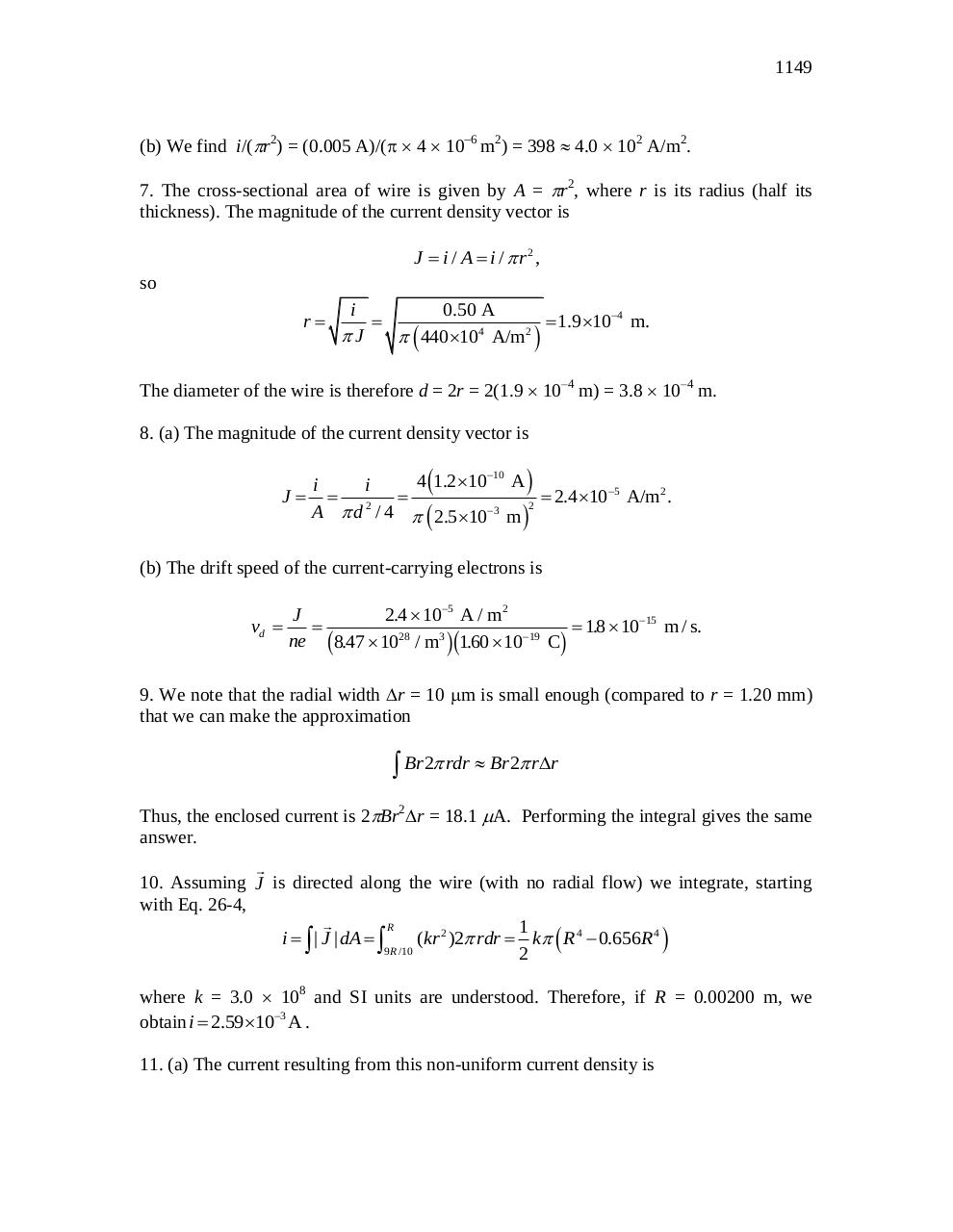# chapter 26.pdfPage 1 2 34526

#### Text preview

1149

(b) We find i/(r2) = (0.005 A)/(4 106 m2) = 398  4.0  102 A/m2.
7. The cross-sectional area of wire is given by A = r2, where r is its radius (half its
thickness). The magnitude of the current density vector is
J  i / A  i /  r2,

so

i
0.50 A

 1.9 104 m.
4
2
J
  440 10 A/m 

r

The diameter of the wire is therefore d = 2r = 2(1.9  10–4 m) = 3.8  10–4 m.
8. (a) The magnitude of the current density vector is

4 1.2 1010 A 
i
i
J  2 
 2.4 105 A/m2 .
2

3
A  d / 4   2.5 10 m 
(b) The drift speed of the current-carrying electrons is

vd 

J
2.4  105 A / m2

 18
.  1015 m / s.
19
ne 8.47  1028 / m3 160
.  10 C

c

hc

h

9. We note that the radial width r = 10 m is small enough (compared to r = 1.20 mm)
that we can make the approximation

 Br 2 rdr  Br 2 rr
Thus, the enclosed current is 2Br2r = 18.1 A. Performing the integral gives the same